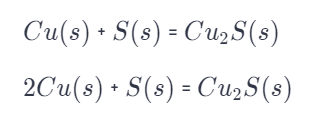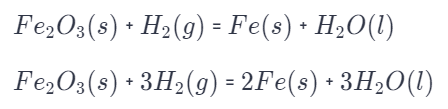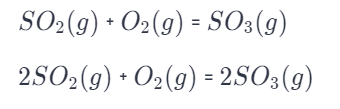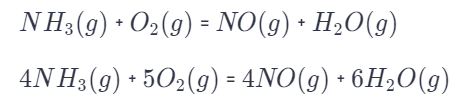# Solid Copper Reacts With Solid Sulfur To Form Solid Copper(I) Sulfide.

We thoroughly check each answer to a question to provide you with the most correct answers. Found a mistake? Let us know about it through the REPORT button at the bottom of the page.

Write the balanced chemical equation for each reaction.

a. Solid copper reacts with solid sulfur to form solid copper(I) sulfide.

b. Solid iron (III) oxide reacts with hydrogen gas to form solid iron and liquid water.

c. Sulfur dioxide gas reacts with oxygen gas to form sulfur trioxide gas.

d. Gaseous ammonia (NH3) reacts with gaseous oxygen to form gaseous nitrogen monoxide and gaseous water.

Contents

A) 2Cu(s) + S(s) = Cu2S(s)

B)  Fe2O3(s) + 3H2(g) = 2Fe(s) + 3H2O(l)

C) 2SO2(g) + O2(g) = 2SO3(g)

D) 4NH3(g) + 5O2(g) = 4NO(g) + 6H2O(g)

## Explanation

The first equation is unbalanced because on the right side of the equation is 2 atoms of copper, and on the left is one.

The second one is balanced.The second one is a balanced equation. There are 2 atoms of Fe, 6 of H, and 3 of O.The second one is a balanced equation. There are 2 atoms of S and 6 atoms of O.There are 4 atoms of N, 10 of O, and 12 of H in a balanced equation.